# Perfect ring

(diff) ← Older revision | Latest revision (diff) | Newer revision → (diff)
The following properties of a ringare equivalent: 1)is a left perfect ring; 2) every set of pairwise orthogonal idempotents ofis finite, and every non-zero right-module has a non-zero socle; 3)satisfies the minimum condition for principal right ideals; 4)satisfies the minimum condition for finitely-generated right ideals; 5) every right-module satisfies the minimum condition for finitely-generated submodules; 6) the Jacobson radical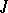ofvanishes on the right (that is, for any sequence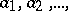of elements ofthere is an integersuch that the product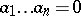), and the quotient ringis Artinian semi-simple; 7) every flat left-module is projective; 8)contains idempotents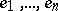such that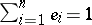,for, andis a local ring for every; 9) every left-module satisfies the maximum condition for cyclic submodules; 10) for everyevery left-module satisfies the maximum condition for-generated submodules; and 11) every projective left-module has a decomposition with respect to which every direct summand has a complement (see Krull–Remak–Schmidt theorem).
A ring of matrices over a perfect ring is perfect. Idempotent ideals of a perfect ring are generated by idempotents that are central modulo the radical. A group ring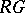(see Group algebra) is perfect if and only ifis a perfect ring andis a finite group. The endomorphism ring of an Abelian groupis perfect only whenis the direct sum of a finite group and finitely many copies of the additive group of rational numbers. Local perfect rings are characterized by the fact that any linearly independent subset of any free left module over it can be extended to a base. The following properties are also equivalent: a)is a perfect ring and all its quotient rings are self-injective (cf. Self-injective ring); b) every quotient ring ofis quasi-Frobenius (cf. Quasi-Frobenius ring); c) every quotient ring ofhas a cogenerator; and d)is uniserial (cf. Uniserial ring).